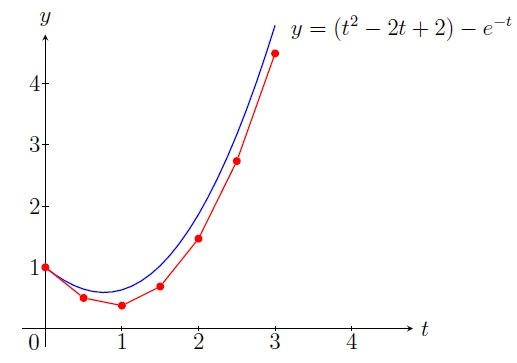Numerical Analysis Home

## Euler's Method

Introduction to IVP: In this chapter we numerically solve the following IVP (initial value problem): $\frac{dy}{dt}=f(t,y),\,a\leq t\leq b,\, y(a)=c \;\;\;\;\;\;\;\;\;\;\;\; (1)$ Instead of finding $$y=y(t)$$ on $$[a,b]$$, we break $$[a,b]$$ into $$n$$ subintervals $$[t_0,t_1],[t_1,t_2],\ldots,[t_{n-1},t_n]$$ and approximate $$y(t_i),\,i=0,1,\ldots,n$$. But if we need $$y(t)$$, it can be approximated by the Lagrange polynomial $$P_n$$ using $$t_0,t_1,\ldots,t_n$$.

Before approximating a solution $$y=y(t)$$, we must ask if the IVP (1) has a solution and it is unique on $$[a,b]$$. The answer is given by the Existence and Uniqueness Theorem:

Theorem. The IVP (1) has a unique solution $$y(t)$$ on $$[a,b]$$ if

1. $$f$$ is continuous on $$D=\{(t,y)\,|\, a\leq t\leq b,\, -\infty< y< \infty \}$$), and

2. $$f$$ satisfies a Lipschitz condition on $$D$$ with constant $$L$$: $|f(t,y_1)-f(t,y_2)|\leq L|y_1-y_2|,\, \text{for all } (t,y_1),(t,y_2)\in D.$

When we approximate $$y(t_i),\,i=0,1,\ldots,n$$ for the unique solution $$y(t)$$, we might commit some round-off errors. So we ask if the IVP (1) is well-posed: a small change in the problem (i.e., small change in $$f,c$$) gives a small change in the solution.

It can be proved that the IVP (1) is well-posed if $$f$$ satisfies a Lipschitz condition on $$D$$. Also note that if $$|f_y(t,y)|\leq L$$ on $$D$$, then $$f$$ satisfies a Lipschitz condition on $$D$$ with constant $$L$$.

Euler's Method: We break $$[a,b]$$ into $$n$$ subintervals $$[t_0,t_1],[t_1,t_2],\ldots,[t_{n-1},t_n]$$ where $$t_i=a+ih$$ and $$h=(b-a)/n$$. The Euler's Method finds $$y_0,y_1,\ldots,y_n$$ such that $$y_i\approx y(t_i),\,i=0,1,\ldots,n$$: \boxed{ \begin{aligned} y_0 &=c\\ y_{i+1} &=y_i+hf(t_i,y_i),\, i=0,1,\ldots,n-1. \end{aligned}}

To justify the iterative formula, use Taylor's theorem on $$y$$ about $$t=t_i$$: $\begin{array}{rrl} &y(t) &= y(t_i)+(t-t_i)y'(t_i)+\frac{(t-t_i)^2}{2}y''(\xi_i)\\ \implies &y(t_{i+1}) &= y(t_i)+(t_{i+1}-t_i)y'(t_i)+\frac{(t_{i+1}-t_i)^2}{2}y''(\xi_i)\\ & &= y(t_i)+hf(t_i,y(t_i))+\frac{h^2}{2}y''(\xi_i)\\ \implies & y(t_{i+1})& \approx y_i+hf(t_i,y_i) =: y_{i+1} \end{array}$

Example. Use Euler's method with step size $$h=0.5$$ to approximate the solution of the following IVP: $\frac{dy}{dt}=t^2-y,\, 0\leq t\leq 3,\, y(0)=1$ Solution. We have $$h=0.5,\, t_0=0,\, y_0=1$$ and $$f(t,y)=t^2-y$$. So $y_{i+1}=y_i+0.5(t_i^2-y_i)=0.5(t_i^2+y_i).$ \begin{align*} t_1&=0+1\cdot 0.5=0.5, &&y_1=0.5(t_0^2+y_0)=0.5(0+1)=0.5\\ t_2&=0+2\cdot 0.5=1, &&y_2=0.5(t_1^2+y_1)=0.5(0.25+0.5)=0.375 \; \text{etc.} \end{align*} $\begin{array}{|c|l|l|} \hline i & t_i & y_i \\ \hline 0&0 &1\\ \hline 1&0.5&0.5\\ \hline 2&1 &0.375\\ \hline 3&1.5&0.6875\\ \hline 4&2 &1.46875\\ \hline 5&2.5&2.734375\\ \hline 6&3 &4.4921875\\ \hline \end{array}$Geometric Interpretation: The tangent line to the solution $$y=y(t)$$ at the point $$(t_0,y_0)$$ has slope $$\displaystyle\left.\frac{dy}{dt}\right]_{(t_0,y_0)}=f(t_0,y_0)$$. So an equation of the tangent line is $y=y_0+(t-t_0)f(t_0,y_0).$ If $$t_1$$ is close to $$t_0$$, then $$y_1=y_0+(t_1-t_0)f(t_0,y_0)=y_0+hf(t_0,y_0)$$ would be a good approximation to $$y(t_1)$$. Similarly if $$t_2$$ is close to $$t_1$$, then $$y(t_2)\approx y_2=y_1+hf(t_1,y_1)$$.

Maximum error: Suppose $$D=\{(t,y)\,|\, a\leq t\leq b,\, -\infty< y< \infty \}$$ and $$f$$ satisfies a Lipschitz condition on $$D$$ with constant $$L$$. Suppose $$y(t)$$ is the unique solution to (1) where $$|y''(t)|\leq M$$ for all $$t\in [a,b]$$. Then for the approximation $$y_i$$ of $$y(t_i)$$ by the Euler's method with step size $$h$$, we have $|y(t_i)-y_i|\leq \frac{hM}{2L}\left[ -1+e^{L(t_i-a)} \right],\, i=0,1,\ldots,n.$

Example. Find the maximum error in approximating $$y(1)$$ by $$y_2$$ in the preceding example. Compare it with the actual absolute error using the solution $$y=(t^2-2t+2)-e^{-t}$$.

Solution. $$f(t,y)=t^2-y \implies |f_y|=|-1|\leq 1=L$$ for all $$y$$. Thus $$f$$ satisfies a Lipschitz condition on $$D=(0,3)\times(-\infty,\infty)$$ with the constant $$L=1$$. Now $y=(t^2-2t+2)-e^{-t} \implies y''= 2-e^{-t}.$ Since $$y'''=e^{-t}>0$$, $$y''$$ is an increasing function and then $|y''|= |2-e^{-t}|\leq 2-e^{-3}=1.95=M\; \text{ for all }t\in [0,3]$ Note $$h=0.5$$, $$t_2=1$$, and $$a=0$$. Thus $|y(1)-y_2|\leq \frac{hM}{2L}\left[ -1+e^{L(t_2-a)} \right]= \frac{0.5\cdot 1.95}{2\cdot 1}\left[ -1+e^{1(1-0)} \right] = 0.83$ Using the solution $$y=(t^2-2t+2)-e^{-t}$$, we get the actual absolute error $|y(1)-y_2|=|(1-e^{-1})-0.375|=0.25.$

Last edited